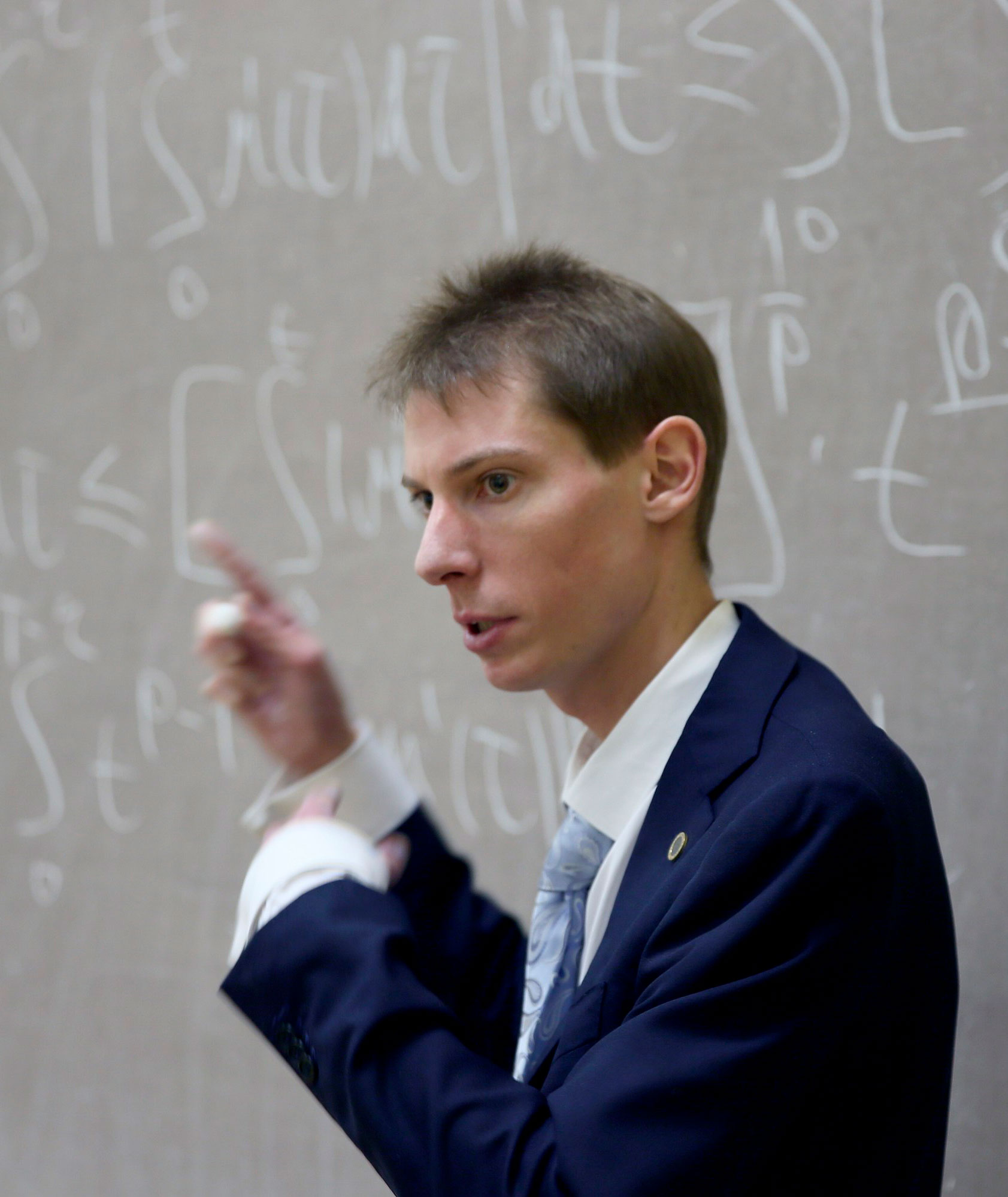﻿ Laboratory site
En / Ru## Kuleshov Alexander Andreevich

E-mail:

Websites:Education:

→ Graduated with honours from the Faculty of Computational Mathematics and Cybernetics of Lomonosov Moscow State University in 2009;

→ In 2012 defended his PhD Thesis "An analytical form of generalized solutions of mixed problems for the wave equation in the case of nonlocal boundary conditions and discontinuous coefficients" under the supervision of the academician V.A. Il'in.

### Research Interests:

Real and functional analysis, function theory, partial differential equations.

### Honors and Awards:

Golden RAS Medal for Russian students in mathematics, 2009.

### Publications (recent):

2023

→ M. D. Kovalev, A. A. Kuleshov, "On Properties of Continuous Monotone Functions", Math. Notes, 113:6 (2023), 874–878, DOI.

2021

→ A. Kuleshov, "A Remark on the Change of Variable Theorem for the Riemann Integral", Mathematics, 9:16 (2021), 1899, DOI.

2020

→ A. Kuleshov, "The Various Definitions of Multiple Differentiability of a Function $$f\colon \mathbb{R}^n → \mathbb{R}$$", Mathematics, 8:11 (2020), 1946, 6 pp, DOI.

2019

→ A. A. Kuleshov, "Continuous Sums of Ridge Functions on a Convex Body with Dini Condition on Moduli of Continuity at Boundary Points", Analysis Mathematica, 45 (2019), 335-345, DOI.

2018

→ S. V. Konyagin, A. A. Kuleshov, V. E. Maiorov, "Some Problems in the Theory of Ridge Functions", Proc. Steklov Inst. Math., 301 (2018), 144–169, DOI.

→ A. A. Kuleshov, I. S. Mokrousov, I. N. Smirnov, "Solvability of mixed problems for the Klein–Gordon–Fock equation in the class $$L_p$$ for $$p≥1$$", Differ. Equ., 54:3 (2018), 330–334, DOI.

2017

→ A. A. Kuleshov, "Continuous Sums of Ridge Functions on a Convex Body and the Class VMO", Math. Notes, 102:6 (2017), 799–805, DOI.